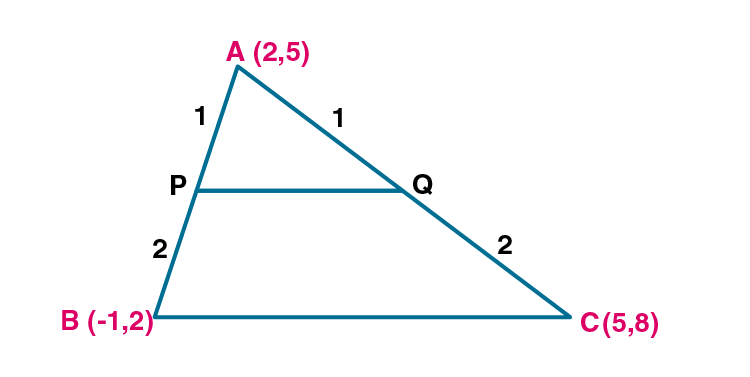Newbie

# A (2, 5), B ( – 1, 2) and C (5, 8) are the vertices of a triangle ABC. P and Q are points on AB and AC respectively such that AP : PB = AQ : QC = 1 : 2. (i) Find the co-ordinates of P and Q. (ii) Show that PQ = 1/3 BC

• 1

An Important Question of class 10 Based on Section Formula Chapter of M.L Aggarwal for ICSE BOARD.
Here given three points are the vertices of a triangle ABC
This is the Question Number 32, Exercise 11 of M.L Aggarwal.

Share

1.(i) Given vertices of the ABC are A(2,5), B(-1,2) and C(5,8).

P and Q are points on AB and AC respectively such that AP:PB = AQ :QC = 1:2.

P(x,y) divides AB in the ratio 1:2.

x1= 2, y1 = 5

x= -1, y2 = 2

m:n = 1:2

By section formula, x = (mx2+nx1)/(m+n)

x = (1×-1+2×2)/(1+2)

x = (-1+4)/(3)

x = 3/3 = 1

By section formula, y = (my2+ny1)/(m+n)

y = (1×2+2×5)/(1+2)

y = (2+10)/(3)

y = 12/3 = 4

Co-ordinates of P are (1,4).

Q(x,y) divides AC in the ratio 1:2.

x1= 2, y1 = 5

x= 5, y2 = 8

m:n = 1:2

By section formula, x = (mx2+nx1)/(m+n)

x = (1×5+2×2)/(1+2)

x = (5+4)/(3)

x = 9/3 = 3

By section formula, y = (my2+ny1)/(m+n)

y = (1×8+2×5)/(1+2)

y = (8+10)/(3)

y = 18/3 = 6

Co-ordinates of Q are (3,6).

(ii) By distance formula, d(PQ) = √[(x2-x1)2+(y2-y1)2]

Points are P(1,4) and Q(3,6).

So x1= 1, y1 = 4

x= 3, y2 = 6

d(PQ) = √[(x2-x1)2+(y2-y1)2]

d(PQ) = √[(3-1)2+(6-4)2]

d(PQ) = √[(2)2+(2)2]

d(PQ) = √(4+4)

d(PQ) = √8 = 2√2 ..(i)

By distance formula, d(BC) = √[(x2-x1)2+(y2-y1)2]

Points are B(-1,2) and C(5,8).

So x1= -1, y1 = 2

x= 5, y2 = 8

d(BC) = √[(x2-x1)2+(y2-y1)2]

d(BC) = √[(5-(-1))2+(8-2)2]

d(BC) = √[(6)2+(6)2]

d(BC) = √(36+36)

d(BC) = √72 = √(36×2) = 6√2 ..(ii)

BC/3 = 6√2/3 = 2√2 = PQ

PQ = 1/3 BC.

Hence proved.

• 0